Adding and Subtracting Fractions Test
Name *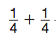1. Find the sum. *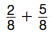2. Find the sum. *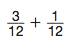3. Find the sum. *
The table shows the distances David hiked on each day of a camping trip.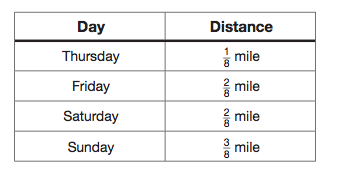4. How far in total did David hike on Thursday and Friday ? *
5. How far in total did David hike on Friday and Saturday? *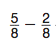6. Find the difference. *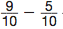7. Find the difference. *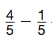8. Find the difference. *
9. Of the fifth graders at Oak Elementary, 6/8 are in band. What fraction of the fifth-grade students are NOT in band? *
10. Joan counted that 2/10 of her jelly beans were red. Dean counted that 6/10 of his jelly beans were red. How much greater a fraction of Dean’s jelly beans were red? *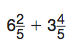11. Find the sum. *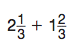12. Find the sum. *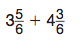13. Find the sum. *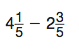14. Find the difference. *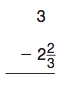15. Find the difference. *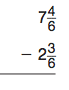16. Find the difference. *